LAMINATED RESERVOIR basicS
Porosity and water saturation in laminated shaly sands, and in other cases of anisotropic reservoirs, are a special case, not amenable to conventional petrophysical solutions. Isotropic reservoirs are those in which the physical properties are the same regardless of the direction of measurement. Anisotropic reservoirs have one or more properties that vary with direction.

The best known anisotropic property is resistivity, which can vary by a factor of 100 or more, depending on whether the measurement is made parallel to the bedding or perpendicular to it. This is the situation that exists in most so-called "low resistivity pay zones". These are usually laminated shaly sands but can also be sandstones or carbonates with thinly bedded variations in porosity. In resistivity log analysis, anisotropy is present when the bedding is thinner than the tool resolution and is sometimes described as a "thin-bed" problem.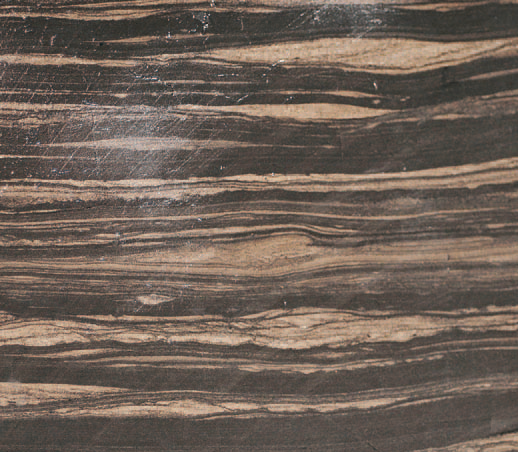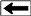A core photo, roughly full size, of a short interval of the Second White Specks, a laminated sand tight gas or tight oil play in Alberta. Some sand lenses are as thin as a pencil line.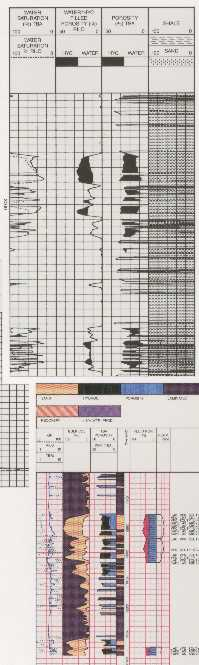Rocks of this type are called transverse isotropic; there is little horizontal anisotropy, so resistivity differs between only two axes - vertical and horizontal. Channel sands with significant cross bedding and other linear depositional features could be anisotropic on all three axes.

There are no logs that measure resistivity in 3 orthogonal axes at the same time. The newest induction logs measure horizontal and vertical resistivity (directions relative to tool axis). Azimuthal laterologs read in eight directions (perpendicular to the tool axis) and could be used to look for horizontal anisotropy in semi-vertical wells.

A modern thin bed log, called the TBRt by Baker Hughes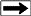The newest thin bed tool is described as a thin bed Rt tool. It is a microlaterolog type of device with a bed resolution of 5 cm and a depth of investigation between 30 and 50 cm (12 to 20 inches), about 2 to 3 times deeper than earlier microlaterologs. If invasion is shallow, the resistivity approaches a deep resistivity measurement. This is very useful in laminated shaly sands where the laminae are relatively thick.

Thin bed Rt log used to shape final log analysisOther thin bed logging tools are the microlog, microlaterolog, proximity log, and micro spherically focused log. These tools measure 3 to 12 centimeters of rock but have a depth of investigation of similar dimensions. In some laminated sands, these tools can be used to determine net to gross sand ratio.

The electromagnetic propagation log measures in the order of 6 cm but it is a porosity and shale indicator tool, not a deep resistivity tool. Some sonic logs can be run with a 15 cm (6 inch) bed resolution.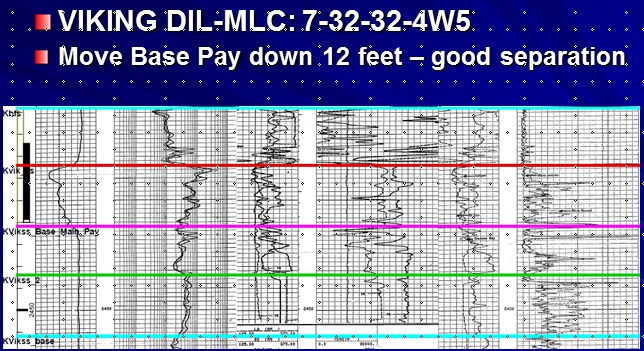Example of conventional resistivity and density neutron log in laminated porosity, with microlog showing numerous very thin tight streaks. Positive separation between the two microlog curves shows porous intervals.

The resistivity microscanner can see beds as thin as 0.5 cm and fractures as thin as 1 micron. The acoustic televiewer can resolve beds to 1 or 2 cm. Accurate net to gross ratios can be determined, but again the resistivity of the sand fraction beyond the invaded zone cannot be determined from these tools.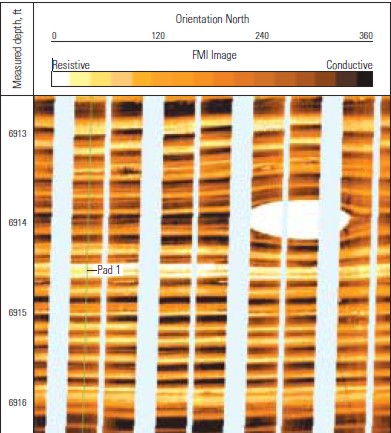None of the tools listed above provide a useful deep resistivity value when laminations are thinner than the tool resolution, so unconventional log analysis models are needed.

While laminated shaly sands are best known, laminated porosity is also a problem for log analysts. The Bakken and Montney reservoirs in Canada are good examples. The illustrations below give a clear example of how porosity logs and analysis results smooth out the porosity variations, which in turn smooth out the saturation and permeability answers. The latter is especially critical, since productivity estimates for laminated reservoirs can be seriously under-estimated because the high permeability streaks tend to be ignored.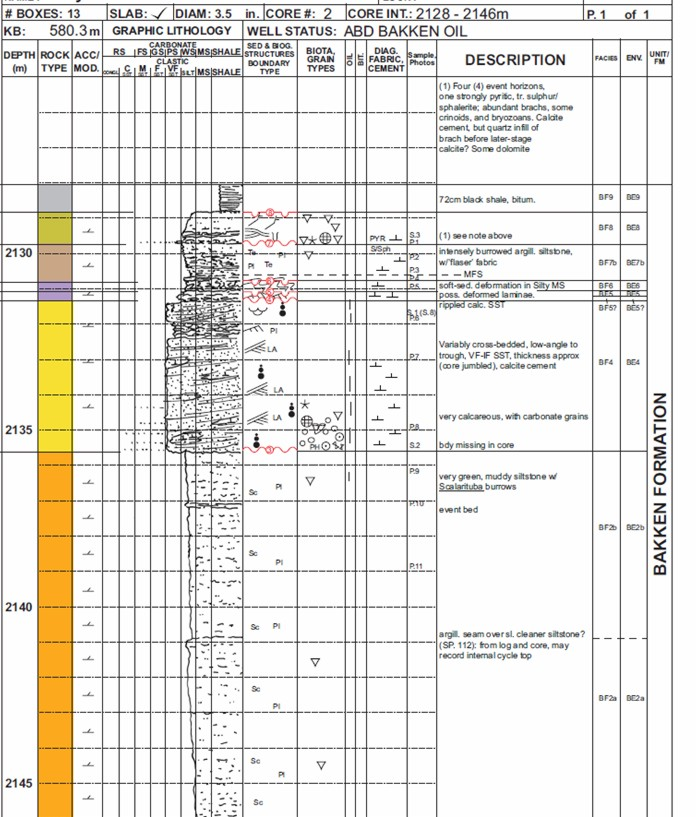Core description log in a laminated Bakken sand. Upper half of interval is highly laminated, lower half has thicker beds. See plot of core data below. (Illustration courtesy Graham Davies Geological Consultanting)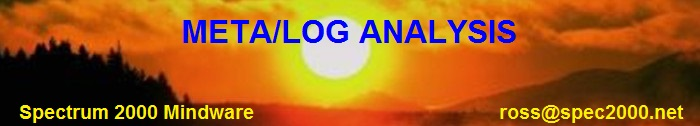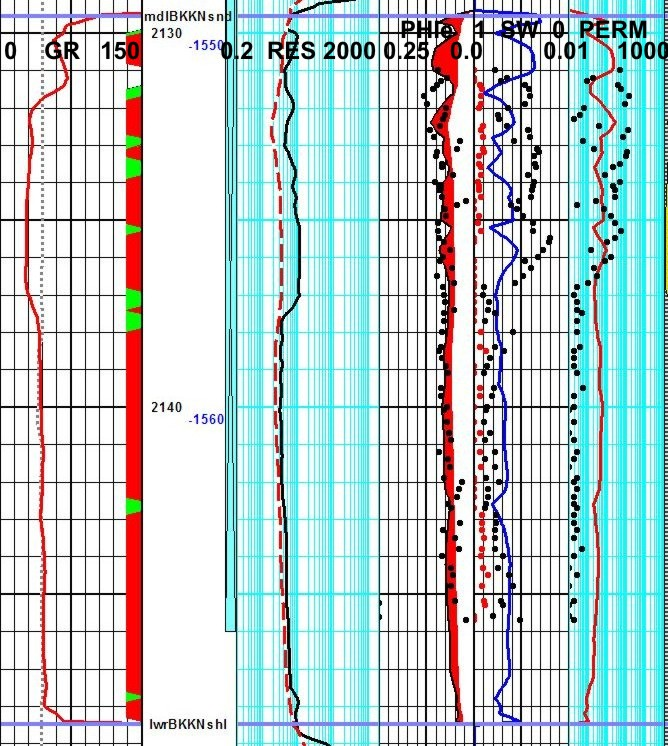Closely spaced core samples demonstrate laminated nature of Bakken sand, compared to the running average created by well logs. Distinct coarsening upward and fining upward sequences can be seen in the upper half of core (grid lines are 1 meter). The lower half of the cored interval is less laminated, so porosity and permeability variations are smaller. Longer running average on resistivity log makes water saturation even more difficult to assess and comparison to core is worse than for porosity and permeability
Logs and core are for same well as core description shown above.Resistivity in Anisotropic Reservoirs
The problem lies in how resistivity logs average laminations that are thinner than the tool resolution. Most logs average the data in a linear, thickness weighted fashion, but induction and laterologs average conductivity and then convert it to resistivity. In shaly sands, the conductivity of the shale laminations is usually much higher than the gas or oil sand laminations, the resulting conductivity is high (low resistivity). This makes the zone look like a poor quality reservoir, maybe so poor that it will not be tested, thus bypassing considerable oil or gas.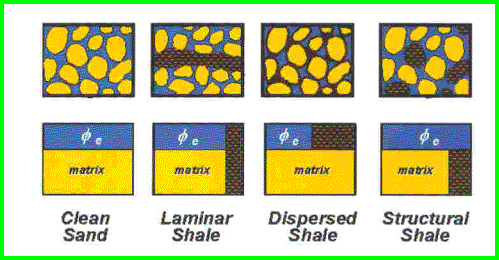The physical model for a laminated shaly sand compared to a clean sand and conventional shaly sands. The high conductivity of the shale lamination (black shading) strongly influences the net conductivity measured by resistivity tools.A similar problem exists in laminated porosity. The low porosity laminations have higher water saturation than oil or gas bearing higher porosity laminations. The measured resistivity of the laminated hydrocarbon bearing reservoir is often close to the truth, but the calculated water saturation of water zones may be misleading.To illustrate the simplest case, assume a laminated shaly sand sequence with shale laminations equal in thickness to the sand laminations. This gives a shale volume (Vsh) averaged over the interval of 50%. Assume the porosity and resistivity values are as shown at the right.

The average total porosity in this example is 0.20; the average effective porosity is only 0.10 – that’s what the density neutron logs see. The actual porosity in the sand fraction is 0.20 but conventional log analysis cannot tell us that.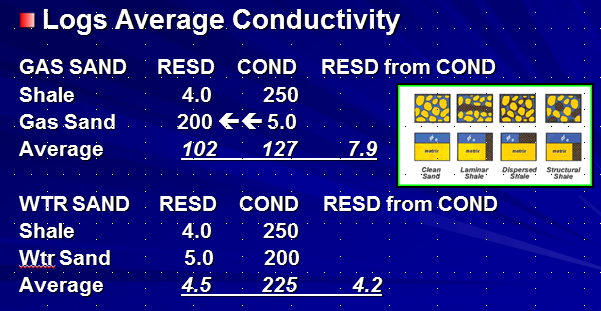The effect of laminations on resistivity is even more serious because the logs really measure conductivity, not resistivity. Again assuming a 50:50 mix of sand and shale laminations, the average conductivity in the illustration at the left is 127 mS, which translates to 7.9 ohm-m.

SO: the average of 4 ohm-m and 200 ohm-m is a little less than 8 ohm-m - pretty scary, but that is what real induction and laterologs do!

To get a good answer for water saturation using an Archie type method, you need to use the 200 ohm-m of the sand fraction (not the measured value of 7.9) with the sand fraction porosity of 0.20 (not the measured value of 0.10).

The lower part of the previous illustration shows the calculation for a laminated water sand. The error in measured resistivity is small, but the resistivity contrast between a water zone and a hydrocarbon zone is small – less than 2:1. The rule of thumb for detecting hydrocarbons is usually 3:1 or more.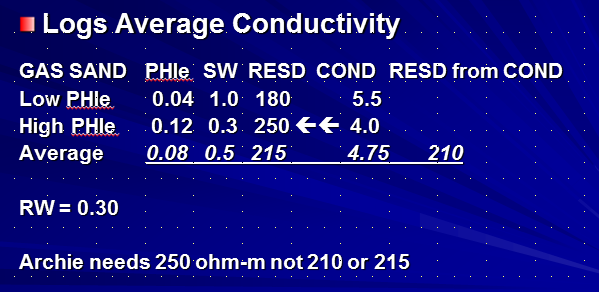The case of laminated porosity is slightly different. The resistivity contrasts are smaller than the laminated shaly sand case. The resistivity of the higher porosity streaks with low water saturation may be close to that of the low porosity streak with higher water saturation. But water zones may look pretty resistive, again giving misleading water saturation.

In this example, the measured resistivity for a 50:50 mix of 4 and 8% porosity laminations in a clean sand is 210 ohm-m, very close to the average of the resistivity values assumed for the two rock types. However, we need to use the 250 ohm-m resistivity of the good quality sand for the saturation calculation, along with the 0.12 porosity to understand the quality of the reservoir. Using the average resistivige and porosity seen by logs will be very misleading.

Modeling laminated shaly sands or laminated porosity with a spreadsheet is the only way to understand the resistivity response and resulting water saturation – usually counter-intuitive, always surprising. A spreadsheet for these models is available as a free download on my website at .3-D Induction logs
Some newer induction logging tools provide a vertical conductivity measurement as well as the usual horizontal measurement. If the beds are still parallel to the horizontal induction log signal, the vertical induction signal will give an average of the resistivity of the beds instead of averaging the conductivity. This is because the normal induction averages the beds in a parallel electrical circuit and the vertical induction sees a series circuit.

Assume a laminated shaly sand with horizontal bedding, a vertical borehole, and a logging tool that can measure both vertical and horizontal conductivity:
1. CONDhorz = VSHavg * CONDshale + (1 - VSHavg) * CONDsand
2. RESvert = VSHavg * RESshale + (1 - VSHavg) * RESsand
3. REShorz = 1000 / CONDhorz
4. CONDvert = 1000 / RESvert
5. AnisRatio = RESvert / REShorz
OR 5. AnisRatio = CONDhorz / CONDvert
6. AnisCoef = AnisRatio ^ 0.5

Where:
AnisRatio = anisotropic ratio
AnisCoef = anisotropic coefficient
CONDhorz = horizontal conductivity (mS/m)
CONDvert = vertical conductivity (mS/m)
CONDsand = sand lamination conductivity (mS/m)
CONDshale = shale lamination conductivity (mS/m)
REShorz = horizontal resistivity (ohm-m)
RESvert = vertical resistivity (ohm-m)
RESsand = sand lamination resistivity (ohm-m)
RESshale = shale lamination resistivity (ohm-m)
VSHavg = shale lamination volume within the interval measured by the logging tool (fractional)

Equations 5 and 6 are as defined by Schlumberger in 1934. Some authors invert the equations so the coefficient is less than or equal to 1.0.

Equations 1 and 2 can be solved simultaneously for any two unknowns if the other parameters are known or computable. For example, we can solve for RESsand and RESshale if RESvert and REShorz are measured log values and VSHavg is computed from (say) the gamma ray log over an interval. Alternatively, we can solve for RESsand and VSHavg if we assume RESshale = RSH from a nearby thick shale:
8. CONDsand = CONDvert * (CONDshale - CONDhorz) / (CONDshl - CONDvert)
9. VSHavg = (CONDhorz - CONDsand) / (CONDshale - CONDsand)

If you prefer to think in Resistivity terms:
10.
RESsand = REShorz * (RESvert - RESshale) / (REShorz - RESshl)
11.
VSHavg = (RESsand - RESvert) / (RESsand - RESshale)

RESsand is then used in Archie's water saturation equation, along with porosity from core or from a laminated sand porosity method, for example:
12: PHINsand = (PHIN - VSHavg * PHINSH) / (1 - VSHavg)
13: PHIDsand = (PHID - VSHavg * PHIDSH) / (1 - VSHavg)
14: PHIsand = (PHINsand + PHIDsand) / 2
15: SWsand = (A * RW@FT / ((PHIsand^M) * RESsand))^(1/N)

Where:
PHINsand = neutron porosity of a sand lamination
PHIN = neutron log reading in the laminated sand
PHINSH = neutron shale value in a nearby thick shale
PHIDsand = density porosity of a sand lamination
PHID = density log reading in the laminated sand
PHIDSH = density shale value in a nearby thick shale

PHIsand = effective porosity of a sand lamination
SWsand = effective water saturation of a sand lamination
RW@FT = water resistivity at formation temperature (ohm-m)
A, M, and N = electrical properties of a sand lamination

Equations 10 through 15 can be plotted versus depth, but this may be misleading since only some of the interval has the porosity and water saturation that is displayed – some of the reservoir interval is nearly pure shale. Oil or gas in place must be adjusted by the net to gross ratio based on the average shale volume:
16: Net2Gross = (1 – VSHavg)

17: NetSand = (1 – VSHavg) * GrossSand

Vertical resistivity logs are still very rare, but are the tool of choice for laminated shaly sands. An example is shown below. Notice the large difference between Rv and Rh on the raw log and the difference in Sw on the computed log.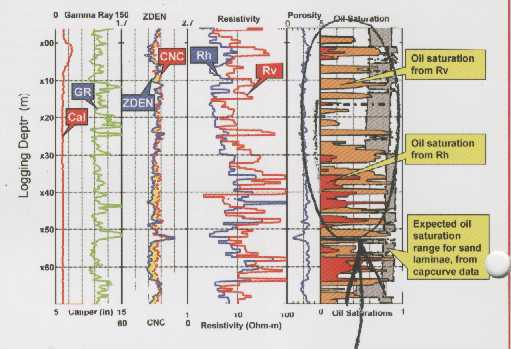Example of vertical and horizontal resistivity in laminated shaly sand3-D Induction logs IN DIPPING BEDS
The example given above involved a laminated shaly sand with bedding perpendicular to the borehole axis (horizontal bedding, vertical borehole). When beds dip relative to the borehole, the situation becomes more complicated. The relative dip is the important factor and takes a bit of thought when the borehole is not vertical.

Dipmeter results are presented as true dip angle and direction relative to a horizontal plane and true north. To obtain dip and direction of beds relative to a logging tool in a deviated borehole, you need the borehole deviation and direction from a deviation survey. This is often obtained at the same time as the dipmeter, but may come from some other deviation survey, either continuous or station by station. You need to rotate the true dips into the plane perpendicular to the borehole to get the final relative dip.

For a conventional induction log, the apparent conductivity is:
18. CONDlog = ((CONDhorz * cos(RelDip))^2 + CONDvert * CONDhorz * (sin(RelDip))^2)^0.5

Where:
CONDlog = conductivity measured by a log in an anisotropic rock (mS/m)
ReLDip = formation dip angle relative to tool axis

When relative dip is 0 degrees (horizontal bed, vertical wellbore), the conventional log reads CONDhorz, as we know it should. However, if relative dip is 90 degrees, as in a horizontal hole in horizontal laminated sands, the log reading is (CONDhorz * CONDvert) ^0.5. This is a surprise, as we might have expected the tool to measure CONDvert.

If two deviated wells are logged through the same formation (at considerably different deviation angles), two equations of the form of equation 18 can be formulated and solved for CONDhorz and CONDvert. RESsand and VSHavg can then be calculated as in equations 10 and 11.AlternAte MODELS – Laminated Shaly SandS
In the absence of a vertical resistivity measurement, we can make some assumptions and use a non-conventional analysis model. These models do not generate log curves that can be plotted versus depth. Instead, they look at stratigraphically significant layers and generate the average properties for each layer.MODEL 1: An obvious solution is to use the math for the vertical resistivity model (equations 10 through 17 given earlier) with assumed values of RESsand (based on a model of a clean sand) and Vsh (based on the GR log). The results would give an indication of the reservoir quality of the individual layer analyzed. Permeability, pore volume (PV), hydrocarbon pore volume (HPV), and flow capacity (KH) are calculated from the above results, just as for conventional sands, bearing in mind that the results apply only to the NetSand portion of the gross interval. No depth plot would be available as the results apply to the whole layer.MODEL 2: Another model uses rules for finding the rock properties based on shale volume, along with constants derived from core analysis. These empirical rules can be calibrated to core and then used where there is no core data. The PHIMAX porosity equation and Buckles water saturation equation given below are widely used in normal shaly sands where the log suite is at a minimum, and are equally useful in the laminated case:
18: VSHavg = average Vsh from GR or density neutron separation over the layer’s gross interval
19: Net2Gross = (1 - VSHavg) or from core, televiewer, or microscanner
20: NetSand = (1 - VSHavg) * Gross
21: PHIsand = PHIMAX
22: SWsand = KBUCKL / PHIsand
OR 22: SWsand = (A * RW@FT / ((PHIsand^M) * RESsand))^(1/N)

Where:
PHIMAX =  maximum porosity expected in the clean sand laminations
KBUCKL = Buckle’s number, product of porosity times water saturation expected in a clean sand lamination

This model presupposes that the laminated sand is hydrocarbon bearing. Again, permeability, pore volume (PV), hydrocarbon pore volume (HPV), and flow capacity (KH) are calculated from the above results, just as for conventional sands, bearing in mind that the results apply only to the NetSand portion of the gross interval.

The PHIMAX value is the critical factor. If a moderate amount of core data is available for the sand fraction of the laminated sand, this data can be mapped and used to control PHIMAX spatially. RESsand can be assumed from a nearby clean hydrocarbon bearing sand or by inverting the Archie equation with reasonable values of PHIMAX, RW@FT, and SW. KBUCKL is usually in the range 0.035 to 0.060, varying inversely with grain size of the clean sand fraction.

A very minimum log suite can be used, since the only curve required is a gamma ray shale indicator, but only if there are no radioactive elements other than clay. This is not the case in the Milk River, so a minimum log suite will not work here. We have used the minimum suite successfully in laminated shaly sands in Lake Maracaibo.MODEL 3: This model uses the linear log response equation to back-out the clean sand fraction properties from the actual log readings and the shale properties. The response equations are used on the average of the log curves over the gross sand interval. We still assume:
23: VSHavg = average Vsh from GR or density neutron separation over gross interval
24: Net2Gross = (1 - VSHavg) or from core, televiewer, or microscanner
25: NetSand = Gross * Net2Gross
26: PHINsand = (PHINavg – VSHavg * PHINSH) / (1 - VSHavg)
27: PHIDsand = (PHIDavg – VSHavg * PHIDSH) / (1 - VSHavg)
28: PHIsand = (PHINsand + PHIDsand) / 2
29: CONDsand = (CONDavg – VSHavg * 1000 / RESshale) / (1 - VSHavg)
30: RESDsand = 1000 / CONDsand
31: SWsand = KBUCKL / PHIsand
OR 31: SWsand = (A * RW@FT / ((PHIsand^M) * RESDsand))^(1/N)

Where:
XXXXavg = log value averaged over a discreet laminated sand interval, thicker than the tool resolution

This model has the advantage of using fewer arbitrary rules and more log data, including resistivity log data. The critical values are RESshale, PHINSH, and PHIDSH, which are picked by observation of the log above the zone. It can still be calibrated to core by adjusting these parameters. If the Archie water saturation equation is used, it might distinguish hydrocarbon from water. The Buckle’s saturation presupposes hydrocarbons are present.

The layer average PHIDsand and PHINsand can be compared to each other to see if they are similar values – they should be if the parameters are reasonably correct. They could cross over if gas effect is strong enough. Our results showed a 0.02 porosity unit variation on the best behaved wells, indicating that the inversion of the response equations was working well. However, on some intervals in some wells, the results were not nearly so good.Reservoir Quality Indicators frOM Laminated Shaly Sand MODELS
There are a number of ways to assess reservoir quality. In laminated sands. One approach is to correlate first three months or first year production with net reservoir properties from one of the laminated models described above. The following example used Model 3 and is from “Productivity Estimation in the Milk River Laminated Shaly Sand, Southeast Alberta and Southwest Saskatchewan” by E. R. (Ross) Crain and, D.W. (Dave) Hume, CWLS Insite, Dec 2004.

We chose to use the first 8760 hours of production (365 days at 24 hours each) divided by 4 (3 months of continuous production) as our “actual” production figure. This normalizes the effects of testing and remedial activities that might interrupt normal production.

The normalized initial production was correlated with net reservoir thickness, pore volume (PV), hydrocarbon pore volume (HPV), and flow capacity (KH). Correlation coefficients (R-squared) are 0.852, 0.876, 0.903, and 0.906 respectively. The correlation is made using data calculated over the total perforated interval.  Average shale volume was correlated with actual production but the correlation coefficient was only 0.296, although the trend of the data is quite clear. Correlation of actual production versus the various reservoir properties are shown below.

Productivity estimate based on Model 3 results and a log analysis version of the productivity equation can be used as well. The equation is:
32. ProdEst = 6.1*10E-6 * KH * ((PF - PS)^2) / (TF + 273) * FR * 90

Where:
KH = flow capacity (md-meters)
(PF - PS) = difference between formation pressure and surface back-pressure (KPa)
TF = formation temperature (degrees Celsius)
FR = hydraulic fracture multiplier (usually 2.0 to 5.0)

The leading constant takes into account borehole radius, drainage radius, and units conversions, and the constant 90 converts e3m3/day into an estimated 3-month production for comparison to actual. A correlation between estimated and actual 90 day production is shown below, top right. Note that the equation used is a constant scaling of KH, so the correlation coefficient is the same as the KH graph at 0.906.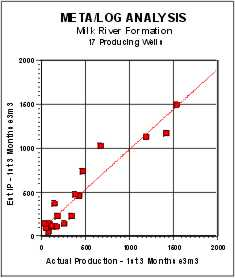Estimated Productivity vs Actual Initial 90 Day Production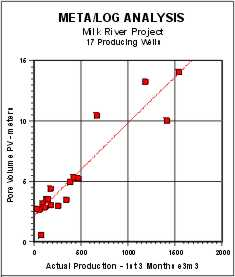Pore Volume (PV) vs Actual Initial 90 Day Production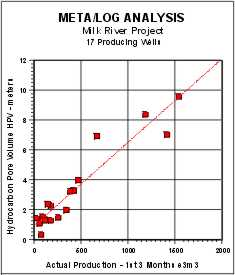Hydrocarbon Pore Volume (HPV) vs Actual Initial 90 Day Production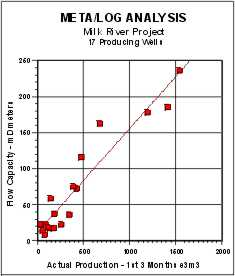Flow Capacity (KH) vs Actual Initial 90 Day Production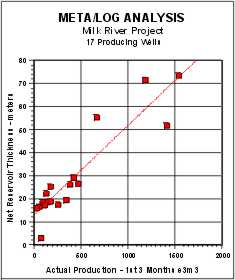Net Sand vs Actual Initial  90 Day Production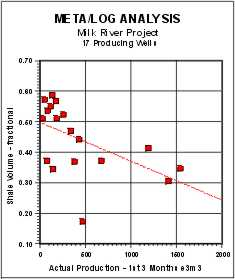Shale Volume (Vsh) vs Actual Initial 90 Day Production

Because a full log suite was available in the 9 wells used for calibration, we have obtained the most likely shale volume (VSHavg) result. The 8 wells held in reserve to test the model also showed very good agreement with initial production. One well that calculated an IP higher than actual can be brought into line with a small tune-up of the shale density parameter.Reservoir Quality from an Enhanced Shale Indicator
Another approach to assessing laminated shaly sands is to generate reservoir quality curves that can be plotted versus depth, to assist in choosing perforation intervals. One such curve is an enhanced GR modified by the resistivity contrast between reservoir and shale values:
33. QualGR = RSH * GR / RESD

Where:
QualGR = enhanced gamma ray quality indicator (API units)
RSH = resistivity of a nearby thick shale (ohm-m)
GR  = gamma ray log reading  (API units)
RESD = deep resistivity log reading  (ohm-m)

This amplifies the shale indicator in cleaner zones (higher net sand) and is scaled the same as the GR curve. A net reservoir cutoff of QualGR <= 50 on this curve was a rough indicator of first three months production, but the correlation coefficient was as poor as for average shale volume. The QualFR cutoff varies from place to place and can be as high as 100 or more. QUALGR does make a useful curve on a depth plot as it shows the best places to perforate when density and neutron data are missing.Reservoir Quality from Hester’s Number
Another quality indicator was proposed in “An Algorithm for Estimating Gas Production Potential Using Digital Well Log Data, Cretaceous of North Montana”, USGS Open File Report 01-12, by T. C. Hester, 1999. It related neutron-density porosity separation and gamma ray response to production, based on the graph in below.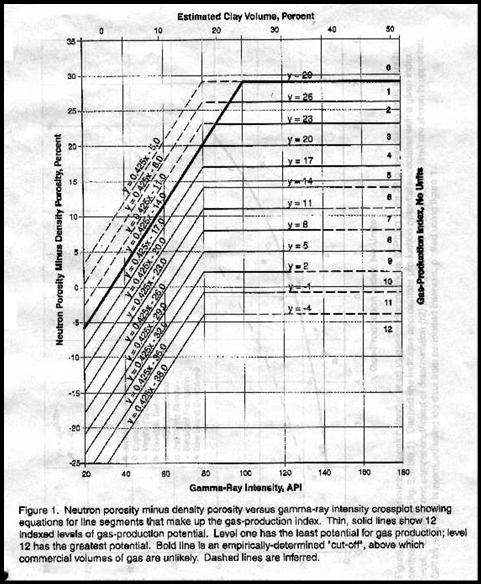Hester’s reservoir quality indicator (Qual1)

This graph is converted to a numerical quality indicator (Qual1) in a complex series of equations that represents predicted flow rate. An Excel and Lotus 1-2-3 spreadsheet for solving this graph is available free on my website at . The equations, as displayed in the Lotus 1-2-3 spreadsheet are as follows:

1: ND_DN = 100 * (PHIN - PHID)
2: E = @IF(ND_DN>(0.425*GR)-14,0,@IF(ND_DN>(0.425*GR)-17,4,
@IF(ND_DN>(0.425*GR)-20,5,@IF(ND_DN>(0.425*GR)-23,6,
@IF(ND_DN>(0.425*GR)-26,7,@IF(ND_DN>(0.425*GR)-29,8,
@IF(ND_DN>(0.425*GR)-32,9,@IF(ND-DN>(0.425*GR)-35,10,11))))))))
3: F = @IF(ND_DN>(0.425*GR)-35,0,@IF(ND_DN>(0.425*GR)-38,11,12))
4: G = @IF(ND_DN>(0.425*GR)-14,0,@IF(ND_DN>29,0,
@IF(ND_DN>26,1,@IF(ND_DN>23,2,@IF(ND_DN>20,3,
@IF(ND_DN>17,4,@IF(ND_DN>14,5,0)))))))
5: H = @IF(ND_DN>14,0,@IF(ND_DN>11,6,@IF(ND_DN>8,7,@IF(ND_DN>5,8,
@IF(ND_DN>2,9,@IF(ND_DN>-1,10,@IF(ND_DN>-4,11,12)))))))
6: I = @IF(E=0,F,E)
7: J = @IF(G=0,H,G)
8: QUAL1 = @IF(GR<80,I,J)

Where:
ND_DN = neutron minus density porosity difference in sandstone units (fractional)
PHID = density porosity sandstone units (fractional)
PHIN = neutron porosity sandstone units (fractional)
GR = gamma ray (API units)
Qual1 = Hester Quality Number (unitless)
E, F, G, H, I, J = intermediate terms

Note that these nested IF statements are slightly different than those originally published by Hester. The changes correct for typographical errors in the original paper.

Hester’s paper only looked at the average quality of a laminated reservoir and did not consider the thickness of a particular quality level. To overcome this, we can use a quality cutoff and obtain a thickness weighted quality and correlate this to actual production, similar to a net pay flag using porosity and saturation cutoffs:
9: IF Qual1 >= X
10: THEN PayFlagQ1 = “ON”
11: AND PayQ1 = PayQ1 + INCR

Where:
X = 4.0 or 5.0
PayQ1 = accumulated pay thickness based on Qual1>= X

A Hester quality of 4.0 or higher reflects reservoir rock that is worth perforating, and gives similar net reservoir thickness as the previous indicators. Graphs showing the correlation of actual production to net reservoir with Qual1 >=5 and >=4 are shown below. The regression coefficients are 0.856 and 0.837 respectively. Although this looks pretty good, the low rate data is clustered very badly and other indicators work better in low rate wells. Some of these wells were not perforated optimally and the Qual1 pay flag is helpful for workover planning.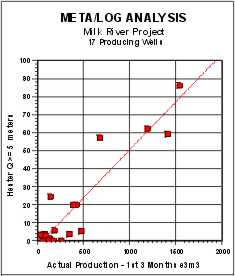Hester Number (Q1 >=5) vs Actual Initial 90 Day Production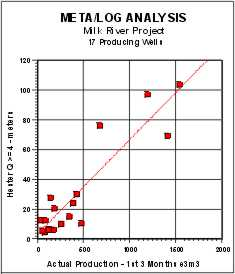Hester Number (Q1 >=4) vs Actual Initial 90 Day Production

###METALOG "/LAM" SPREADSHEET -- LAMINATED SHALY SAND MODELS This spreadsheet calculates laminated shaly sand and laminated porosity models to see the effect of different assumptions on the net resistivity of the interval. It also performs the Hester reservoir quality calculation on individual data points to help assess the best intervals to perforate.

SPR-16 META/LOG LAMINATED SAND CALCULATOR
Model laminated shaly sands and laminated porosity.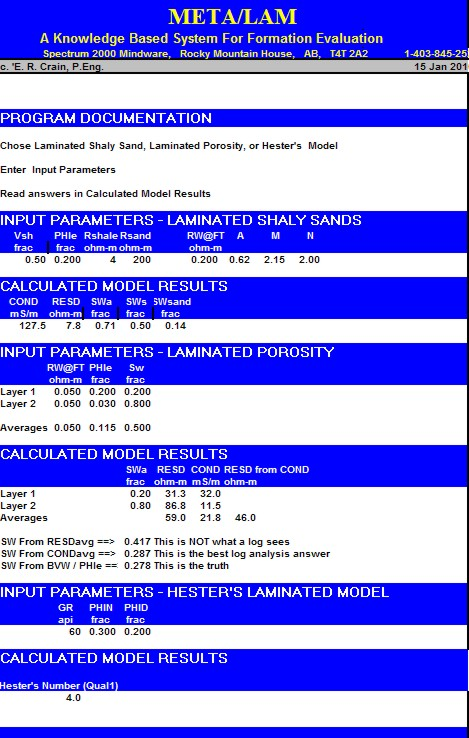Sample output from "META/LAM" spreadsheet for laminated shaly sandstone.LAMINATED SAND EXAMPLE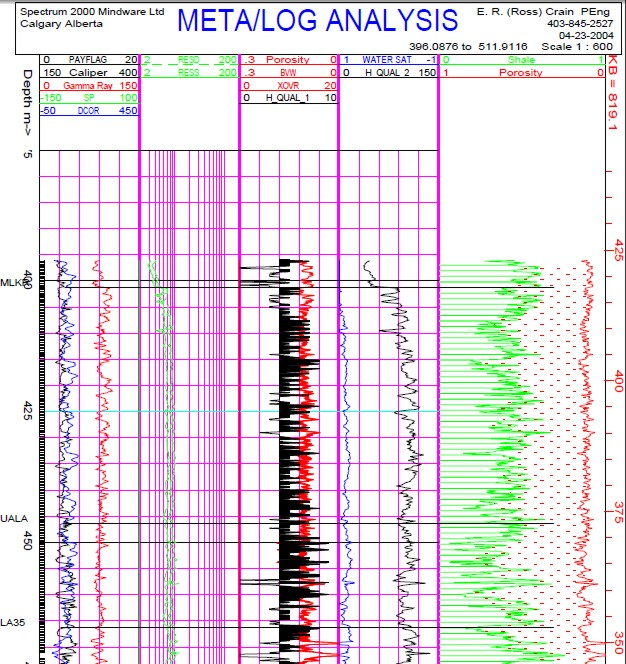Depth plot showing Hester quality factor in Track 3, shaded black where Qual1 >= 4. Zones with Qual1 >= 5 are worth perforating in this area. Enhanced GR quality curve (labeled Qual_2 here) is shown in Track 4. Values of QualGR <= 100 show better quality rock. This is a good well, so nearly all the interval passes these cutoffs. The balance of the analysis is from a conventional shaly sand analysis. Porosity and gas bulk volume (red shading in Track 3) show the best intervals to perforate, but the actual values do not represent the reservoir properties.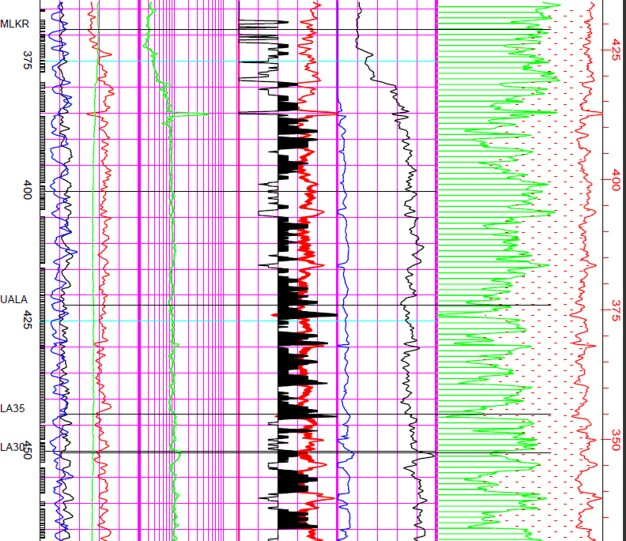Laminated shaly sand example showing poorer quality interval with Qual1 less than 5 that are not worth perforating. Scales and header information are the same as the previous illustration.

Page Views ---- Since 01 Jan 2015
Copyright 2023 by Accessible Petrophysics Ltd.
CPH Logo, "CPH", "CPH Gold Member", "CPH Platinum Member", "Crain's Rules", "Meta/Log", "Computer-Ready-Math", "Petro/Fusion Scripts" are Trademarks of the Author